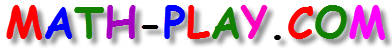#This slope-intercept game has ten multiple choice problems about the slope-intercept form of a linear equation.
Here are some important facts about linear equations that you should know:

The slope-intercept formula of a linear equation is y= mx + b (where m represents the slope and b represents the y-intercept).
The slope is the rise (the vertical change) over the run (the horizontal change).
The y-intercept of a line is the y-coordinate of the point of intersection between the graph of the line and the y-intercept.

You can play this game alone, with a friend, or in two teams. This game is a multi-player game that can be played on computers, Promethean boards, smart boards, iPads, and other tablets. You do not need to install an app to play this game on the iPad. Have fun evaluating algebraic expressions!

The game is based on the following Common Core Math Standards:

CCSS8.F.3
Interpret the equation y = mx + b as defining a linear function, whose
graph is a straight line; give examples of functions that are not linear.,

CCSS8.F.4
Construct a function to model a linear relationship between two
quantities. Determine the rate of change and initial value of the
function from a description of a relationship or from two (x, y) values,
including reading these from a table or from a graph. Interpret the rate
of change and initial value of a linear function in terms of the situation
it models, and in terms of its graph or a table of values.